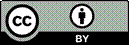Article citation information:

Kirschenstein, M., Krasuski, K., Goś, A. Designating the error of vertical coordinate of aircraft position in the GPS system. Scientific Journal of Silesian University of Technology. Series Transport. 2020, 108, 85-94. ISSN: 0209-3324. DOI: https://doi.org/10.20858/sjsutst.2020.108.8.

Małgorzata KIRSCHENSTEIN, Kamil KRASUSKI, Artur GOŚ3

DESIGNATING THE ERROR OF VERTICAL COORDINATE OF AIRCRAFT POSITION IN THE GPS SYSTEM

Summary. This article presents the results of research concerning the determination of an error of the vertical position of an aircraft during a flight, taking into account, the flight altitude and the values of the ZTD tropospheric product. In particular, this work focuses on the impact of an error in designating the ZTD tropospheric product on the correct determination of a flight altitude. The authors of this work performed a scientific experiment for research data of two flights made by a Cessna 172 around the aerodrome in Dęblin. This article shows the findings of the tropospheric ZTD product specified for the single-frequency SPP positioning method and dual-frequency PPP positioning method. Based on the obtained results, it was discovered that the error of the ZTD tropospheric delay causes an altitude error from 0.08 m at the zenith angle equal to 80o even to 0.79 m at the zenith angle equal to 85o.

Keywords: GPS system, aircraft position, error of vertical coordinate, flight test

1. INTRODUCTION

The emergence of GNSS on-board receivers, on the market, has significantly facilitated the navigation exploitation of the aircraft. The use of GNSS on-board receivers outside the usual navigation functions provides a lot of additional information, for example, connected with specifying atmospheric parameters, that is, the ionospheric and tropospheric delays . Thus, the ionosphere delay constitutes a dispersion part of the atmosphere, whereas the troposphere delay is a neutral part of the atmosphere for GNSS signals . The impact of the ionospheric delay is particularly important in the GNSS differential measurements since it causes vector scaling between the reference station and the on-board GNSS receiver . On the other hand, the tropospheric correction is reflected in determining the aircraft vertical coordinate. An incorrect determination of the aircraft altitude may consequently lead to an air incident or accident. It is, therefore, crucial to conduct scientific research with regard to the determination and monitoring the value of the tropospheric correction in the GNSS satellite measurements in aviation.

The basic equipment of an aircraft is a single frequency GNSS receiver. In this case, the impact of the tropospheric delay is designated using deterministic models . For this reason, the tropospheric delay is added to the geometric distance between the satellite and the receiver in an observation equation in the Single Point Positioning (SPP) code method , similar to an observation equation in GNSS phase measurements. In the SPP method, the tropospheric correction is defined by means of the Slant Troposphere Delay (STD) values. The STD value comprises SHD (Slant Hydrostatic Delay) parameters and SWD (Slant Wet Delay) parameters . The SHD and SWD components are calculated as follows:

-   SHD is determined on the basis of ZHD values (Zenith Hydrostatic Delay) and the mapping function,

-   SWD is determined on the basis of the ZWD value (Zenith Wet Delay) and the mapping function.

The sum of the ZHD and ZWD parameters in its final product is defined as ZTD (Zenith Troposphere Delay .

In case of on-board dual-frequency GNSS receivers, the scheme of designating the tropospheric correction varies. Namely, the ZHD parameter is still determined using the deterministic model, whereas, the ZWD parameter is designated in the stochastic process. To determine the troposphere correction employing the data from the dual-frequency GNSS receiver, the Precise Point Positioning (PPP) method is used . Ultimately, the ZTD tropospheric product is defined as a sum of the ZHD component from the deterministic model and the ZWD component from the stochastic model.

In this paper, the authors present and describe research results concerning the determination of an aircraft vertical error coordinate in the aspect of applying the ZTD tropospheric correction. Furthermore, the authors designate the ZTD parameter based on the single-frequency SPP code method centred on GPS data from an on-board receiver. In addition, the authors of this work also determine the ZTD parameter, using the dual-frequency PPP method based on GPS data derived from an on-board receiver. The difference in the ZTD parameter calculated in the SPP and PPP method allows designating the aircraft vertical coordinate. The calculations are based on real GPS data derived from the on-board GNSS receiver mounted in the Cessna 172. The registered GPS data comes from two air tests performed on the same day (16 June) around the aerodrome in Dęblin.

2. RESEARCH METHODOLOGY

The lack of the aircraft vertical coordinate of the aircraft is determined using the following dependence :(1)

where: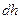- error of vertical coordinate of aircraft (expressed in metres),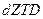- error of Zenith Troposphere Delay (expressed in metres),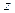- zenith angle (expressed in degrees).

The parameteris determined from a relationship as below: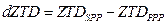(2)

where: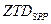- Zenith Troposphere Delay calculated from single-frequency SPP method (expressed in metres),- Zenith Troposphere Delay estimated from dual-frequency PPP method (expressed in metres).

Finally, after a transformation, we receive: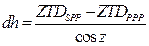(3)

Then, the parameter of the zenith angle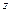can be defined as the completion of the elevation of the angle as shown below:(4)

where:- elevation angle (expressed in degrees).

The relationship from Equation 3 shows the error impact of designating the ZTD parameter upon the accuracy of determining a flight altitude for single-frequency GNSS observations. In Equation 3, the variable parameters are:

-   parameter of the tropospheric delaydetermined in the SPP code method,

-   parameter of the tropospheric delaydetermined in the PPP positioning method,

-   parameter of the zenith angle z,

-   in the analysed case, the parameteris determined from the deterministic model in the SPP code method, whereas the parameterconstitutes the reference value of the ZTD parameter, computed in the PPP measurement technique.

3. THE RESEARCH EXPERIMENT

In the research experiment, the authors designated the aircraft vertical coordinate based on the received data about the ZTD parameter and with regard to the zenith angle. The experimental test was carried out for GPS data coming from two air tests, executed on the same day. The first flight test lasted from 11:58:44 to 12:34:57 in accordance with GPST. The second flight test lasted from 14:31:50 to 15:00:04 in accordance with GPST. Both air tests were conducted by the Cessna 172, on board of which the Topcon HiperPro receiver was installed. In the first air test, the flight altitude of the Cessna 172 reached a maximum of 636 m, whereas in the second test it amounted to 352 m. The vertical flight trajectory in both flight tests is presented in the function of a measurement epoch in Figs. 1 and 2. In the investigations, the value of the ZTD parameter in the SPP code method was designated in the first place. The calculations of the Cessna 172, using the code observations for the SPP method, were made in the RTKLIB v.2.4.2 programme . Furthermore, the ZTD parameter for the SPP code method was determined based on the troposphere model of Saastamoinen. In this case, the ZTD parameter was determined using the deterministic model of the tropospheric delay.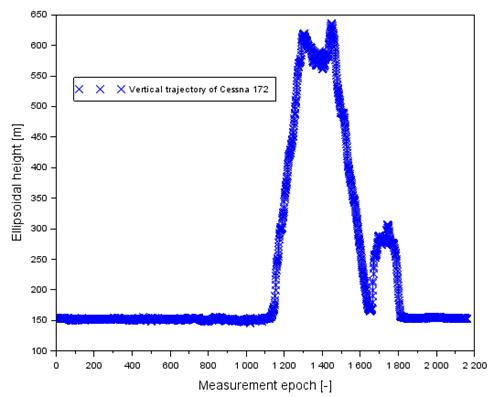Fig. 1. The vertical profile of Cessna 172 in flight no. 1

[Source: based on Scilab software]

In the second one, the authors designated the ZTD based on the PPP measurement technique. The calculations of the position of the Cessna 172, using a dual-frequency GPS code and phase measuring technique in the PPP measurement method was made in the CSRS-PPP v.2.26.0 programme . Furthermore, the ZTD parameter for the PPP measurement technique was determined based on the troposphere VMF model. The ZHD parameter was determined from the deterministic model, whereas the ZWD parameter from the stochastic model of developing GPS code-phase observations.

Thereafter, a comparison of the ZTD value was made in accordance with formula (2) to determine the tropospheric delay error. Finally, the parameter error of the vertical aircraft coordinate was determined in accordance with formula (3). It should be added that the calculation adopted boundary values of the zenith angle from 80o, to 85o, with, with a leap every 1o. The numeric calculations were made in the Scilab v.6.0.0 .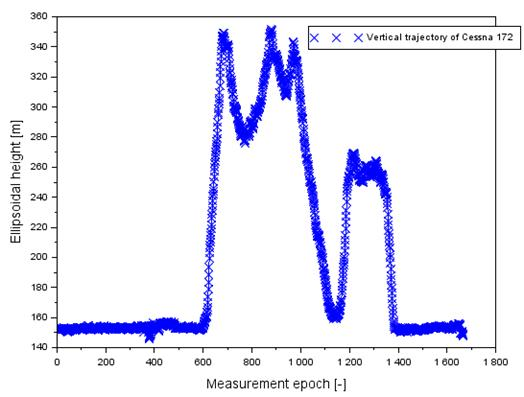Fig. 2. The vertical profile of Cessna 172 in flight no. 2

[Source: based on Scilab software]

4. RESULTS

The obtained results are depicted in Figs. 3 to 6. In the first place, the values of the parameterfor flight no 1 were depicted in Fig. 3. The valuesrange from 0.014 to 0.039 m. Furthermore, the average value of the parameterequals 0.020 m.

Fig. 4 shows the results of designating a flight error of the Cessna 172 during flight no 1. The highest values of the vertical coordinate error are visible for the zenith angle equal to 85o. Then, the parameter takes the values from 0.155 to 0.443 m. In addition, the average value equals 0.220 m and the median value is equal to 0.200 m. For the zenith angle of 84o, the altitude error values range from 0.129 to 0.369 m. Moreover, the average value is 0.191 m, with the median being equal to 0.167 m. For the zenith angle of 83o, the altitude error values range from 0.110 to 0.317 m. In addition, the average value equals 0.164 m, with the median being equal to 0.143 m. For the zenith angle of 82o, the values of altitude error range from 0.097 to 0.277 m. While the average value is 0.144 m, with the median being equal to 0.126 m. For the zenith angle of 81o, the altitude error values are between 0.086 m and 0.247 m. Furthermore, the average value is 0.128 m, with the median being equal to 0.112 m. For the zenith angle of 80o, the altitude error values range from 0.078 to 0.222 m. In addition, the average value is 0.115 m with the median being equal to 0.101 m. Having made an objective assessment of the results in Fig. 4, it is worth stressing that the maximum values are noticeable when the parameter also takes the highest values. On the other hand, when the flight altitude increases, the parameterrises as well.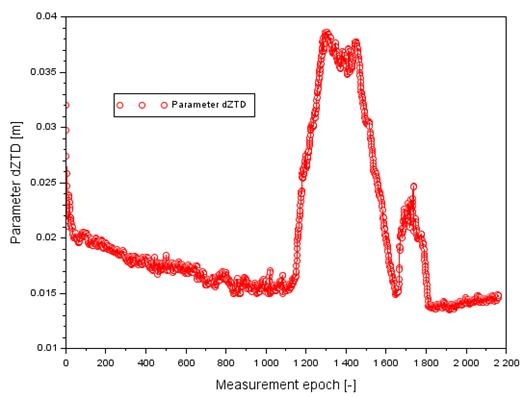Fig. 3. The values of dZTD term in flight no. 1

[Source: based on Scilab software]

In the second place, the values of the parameterfor flight no. 2 were depicted in Fig. 5. The valuesrange from 0.051 to 0.068 m. Furthermore, the average value of the parameterequals 0.060 m.

Fig. 6 shows the results of designating a flight error of the Cessna 172 during flight no 2. The highest error values of the aircraft vertical coordinate are visible for the zenith angle equal to 85o, as well as for flight no. 1. Then, the parametervalue ranges from 0.583 to 0.781 m. In addition, the average valueis 0.685 m with the median being equal to 0.661 m. When the zenith angle equals 84o, the altitude error valuesrange from 0.486 to 0.651 m. Moreover, the average valueis 0.571 m with the median being equal to 0.551 m. Then, the zenith angle is equal to 83o. The altitude error valuesare between 0.417 and 0.558 m. Furthermore, the average valueequals 0.490 m, with the median being equal to 0.473 m.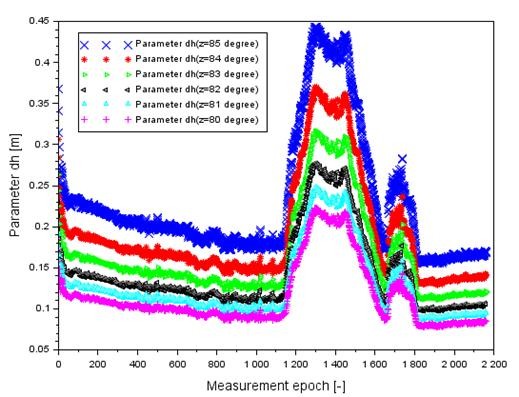Fig. 4. The values of dh parameter in flight no. 1

[Source: based on Scilab software]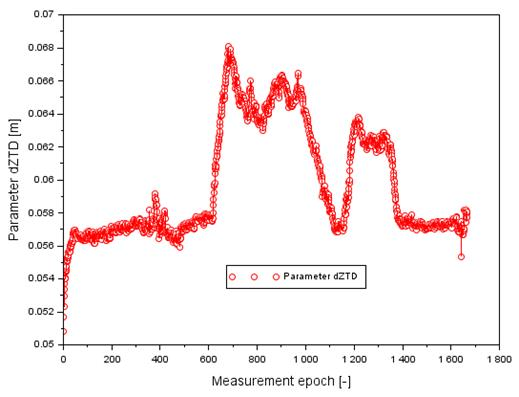Fig. 5. The values of dZTD term in flight no. 2

[Source: based on Scilab software]Fig. 6. The values of dh parameter in flight no. 2

[Source: based on Scilab software]

Then, for the zenith of 82o, the altitude error valuesrange from 0.365 to 0.489 m. In addition, the average valueis equal to 0.429 m, with the median being equal to 0.414 m. Moreover, for the zenith angle equal to 81o, the altitude error valuesare between 0.325 and 0.435 m. Moreover, the average valueis 0.382 m, with the median being equal to 0.368 m. For the zenith angle of 80o, the altitude error valuesrange from 0.293 to 0.391 m. In addition, the average valueis equal to 0.343 m, with the median being equal to 0.332 m. Similar to flight no 1, the values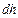are maximum. Also, the parameterhas got the highest value. On the other hand, when the vertical coordinate increases, the parameteralso rises. Based on the results obtained from the flight nos. 1 and 2, it is clear that the impact of the product of tropospheric ZTD is quite significant when determining the component of the vertical position of the aircraft.

5. CONCLUSION

This article presents research findings describing the vertical coordinate error of an aircraft during the execution of experimental flights. This work shows the algorithm of designating the vertical component error of the aircraft position in the function of the ZTD troposphere product error as well as the zenith angle. The scientific experiment was supported by the conducted investigations, in which the research material from two air tests was used. The flights were executed by the Cessna 172 over the aerodrome in Dęblin. This article determines the ZTD tropospheric product on the basis of the deterministic model for the SPP code method. Moreover, the ZTD reference value was defined for the SPP dual-frequency measurement technology. The difference in parameter ZTD has allowed estimating the impact of the tropospheric delay on the designation of real aircraft altitude. Based on the obtained results of the investigations, it was discovered that

-   in test no 1, the error of designating the altitude coordinate of the aircraft equals between 0.078 m for the zenith angle of 80o to 0.443 m for the zenith angle of 85o,

-   in test no 2, the error of designating the altitude coordinate of the aircraft equals between 0.293 m for the zenith angle of 80o to 0.781 m for the zenith angle of 85o,

-   an increase in the error parameter of the ZTD product results in an error increase of designating the altitude coordinate.

The test results can be used in practice for the application of the GPS navigation system in air transport. Moreover, the algorithm presented in this article can also be implemented in the GLONASS system in aviation. The presented work may have a significant impact on research related to the monitoring of the troposphere in air operations and in aircraft navigation.

Acknowledgements

This paper was supported by the Military University of Aviation in the year 2020.

References

1.        Beutler G., I. Bauersima, W. Gurtner, M. Rothacher, T. Schildknechtt, A. Geinger. 1987. Atmospheric refraction and other important biases in GPS carrier phase observations, in atmospheric effects on geodetic space measurements. Monograph 12. Kensington, Australia. P. 15-43.

2.        Bosy J. 2005. „Precise processing of satellite GPS observations in local networks located in mountain areas”. Zeszyty Naukowe Akademii Rolniczej we Wrocławiu 522. ISSN: 0867-7964.

3.        Figurski M. 2001. Określenie wybranych parametrów atmosfery na podstawie permanentnych obserwacji GPS i ich wpływ na dokładność opracowań pomiarów satelitarnych. [In Polish: Determination of selected atmosphere parameters based on permanent GPS observations and their impact on the accuracy of satellite measurements]. ISBN: 83-88442-29-2. Warsaw: WAT.

4.        Kleijer F. 2004. „Troposphere modeling and filtering for precise GPS leveling”. PhD thesis. Department of Mathematical Geodesy and Positioning, Delft University of Technology. Netherlands.

5.        Kutsenko O., S. Ilnytska, V. Konin. 2018. „Investigation of the the residual tropospheric error influence on the coordinate determination accuracy in a satellite landing system”. Aviation 22(4): 156-165.

6.        Sanz Subirana J., Juan Zornoza J. M., Hernández-Pajares M. 2013. GNSS Data Processing. Vol. I: Fundamentals and Algorithms. Publisher: ESA Communications, ESTEC, Noordwijk, Netherlands. ISBN: 978-92-9221-886-7, ESA TM-23/1.

7.        Schaer S. 1999. „Mapping and predicting the Earth’s ionosphere using Global Positioning System”. PhD thesis. Neunundfünfzigster Band. Vol. 59. Zürich, Switzerland.

8.        Schüler T. 2001. “On ground-based GPS tropospheric delay estimation”. PhD thesis. Institute of Geodesy and Navigation, University FAF, Munich Germany.

9.        Takasu T. 2013. RTKLIB ver. 2.4.2 Manual, RTKLIB: an open source program package for GNSS positioning. Available at: http://www.rtklib.com/prog/manual_2.4.2.pdf.

10.    Zhao Q., Y. Yao, W. Yao, Z. Li. 2018. “Real-time precise point positioning-based zenith tropospheric delay for precipitation forecasting”. Scientific Reports 8(7939). DOI: 10.1038/s41598-018-26299-3.

11.    Precise Point Positioning. Available at: https://webapp.geod.nrcan.gc.ca/geod/tools-outils/ppp.php.

12.    Scilab. Available at: https://www.scilab.org/.

Received 11.02.2020; accepted in revised form 24.05.2020